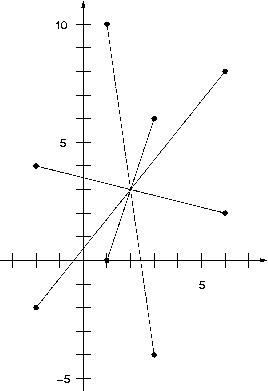Online JudgeProblem SetAuthorsOnline ContestsUser
Web Board
F.A.Qs
Statistical Charts
Problems
Submit Problem
Online Status
Prob.ID:
Register
Authors ranklist
Current Contest
Past Contests
Scheduled Contests
Award Contest
Register
Language:
Center of symmetry
 Time Limit: 1000MS Memory Limit: 65536K Total Submissions: 2617 Accepted: 1173

DescriptionGiven is a set of n points with integer coordinates. Your task is to decide whether the set has a center of symmetry.

A set of points S has the center of symmetry if there exists a point s (not necessarily in S) such that for every point p in S there exists a point q in S such that p-s = s-q.

Input

The first line of input contains a number c giving the number of cases that follow. The first line of data for a single case contains number 1 <= n <= 10000. The subsequent n lines contain two integer numbers each which are the x and y coordinates of a point. Every point is unique and we have that -10000000 <= x, y <= 10000000.

Output

For each set of input data print yes if the set of points has a center of symmetry and no otherwise.

Sample Input

```1
8
1 10
3 6
6 8
6 2
3 -4
1 0
-2 -2
-2 4
```

Sample Output

```yes
```

Source

[Submit]   [Go Back]   [Status]   [Discuss]Home PageGo BackTo top